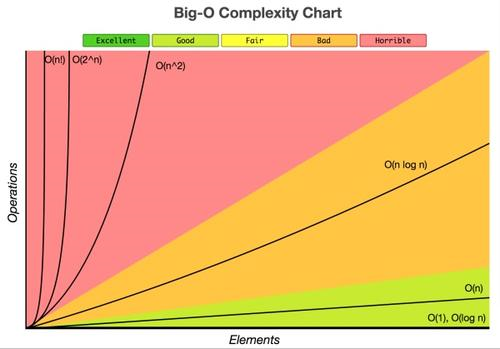# 002算法的描述和分析

### 算法的描述和分析

【1】

• 执行算法所耗费的时间；
• 执行算法所耗费的存储空间；
• 算法应易于理解、易于编码、易于调试等。

• 每条语句的执行时间是：该语句的执行次数和每次执行时间(平均)的乘积
• 一个语句的执行次数称为频度(Frequencry Count)

【2】

1，有穷性

2，确定性

3，输入

4，输出

5，可行性

【3】

``````　　　　for (int j = array.Length - 1; j > 0; j--)   // n-1
{
for (int i = 0; i < j; i++)　　　       //　n(n-1)
{
var sap = array[i];          // n²
array[i] = array[i + 1];    //  n²
array[i + 1] = sap;         //  n²
}
}``````

``T(n) = 4n² + 1``

``````lim T(n)/n² = lim(4n²-1)/n² = 4
n->∞        n->∞

4n²/n² = 4，4
1/n² = m ,而 0< m < 1``````

``````                        var sap = array[i];          // n²
array[i] = array[i + 1];    //  n²
array[i + 1] = sap;         //  n²``````

``````temp = i;
i = j;
j = temp;``````

T(n) = 3，则 T(n) = O(1)。

【4】

``````void func(int n)
{
int i, j, x = 0;
for (i = 0; i < n; ++i) {
for (j = i + 1; i < n; j++){
++x;
}
}
}``````

【5】``````int count = 1;
while(count < n )
count = count * 2;``````

``````n   次数
2   1
4   2
8   3
... ...``````